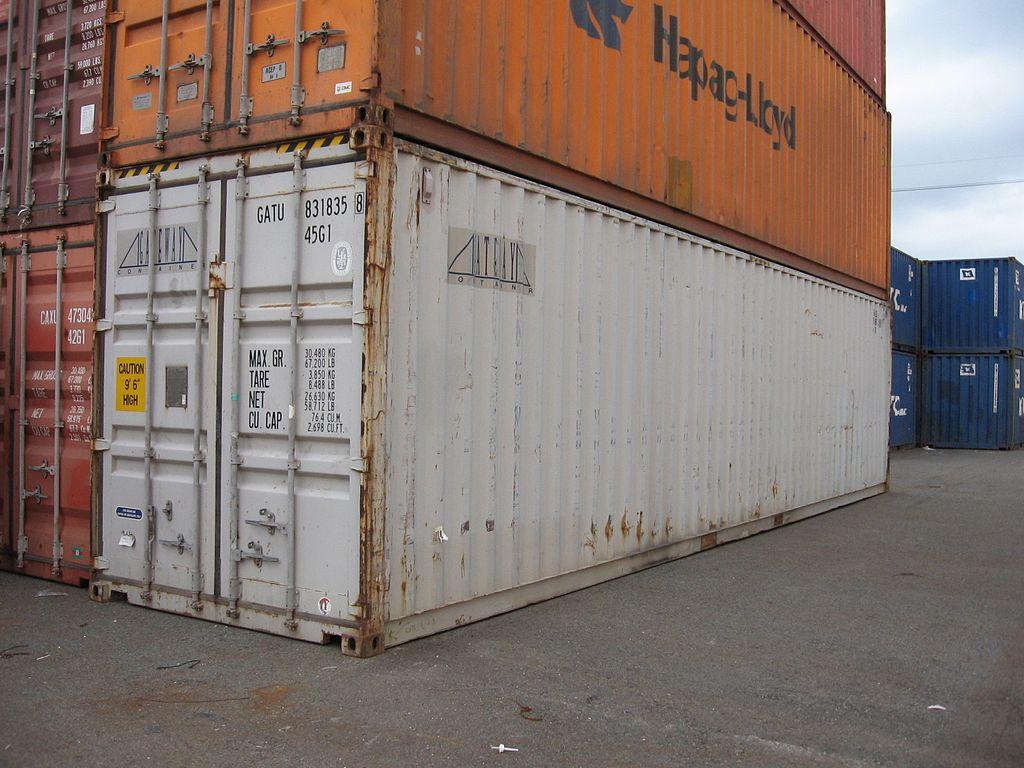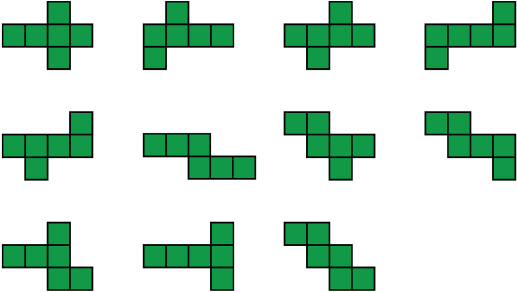# Identifying Nets For Cubes

Student View (Opens in new window)# Lesson Overview

Students explore nets—2-D patterns that can be folded to form 3-D figures. They start by examining several patterns and determining which nets form a cube. Then, they sketch nets for rectangular prisms. They also find the surface area of the rectangular prisms.

ELL: Remind students of the units used to measure area and volume. Use this opportunity to reinforce why square units are used for area (2-D) and cubed units are used for volume (3-D).

# Mathematics

A net is a 2-D pattern that can be folded to form a 3-D figure. In this lesson, the focus is on nets for rectangular prisms. There are many possible nets for any given prism. For example, there are 11 different nets for a cube, as shown below.The surface area of a prism is the area of its net.

# Goals and Learning Objectives

• Identify nets for cubes.
• Sketch the net of a rectangular prism.
• Find the surface area of a rectangular prism.